Russian Academy of Sciences Central Astronomical Observatory at Pulkovo SIXTH US/RUSSIAN SPACE SURVEILLANCE WORKSHOP August 22-26, 2005 Proceedings Edited by P. Kenneth Seidelmann and Victor K. Abalakin St. Petersburg, 2005<<< Contents

### ON A NEW VERSION OF THE ORBIT DETERMINATION METHOD

Alla S. Sochilina

Central Astronomical Observatory at Pulkovo, St.-Petersburg, Russia

Abastumani Astrophysical Observatory, Republic of Georgia, FSU
##### 1. THE METHOD OF DETERMINATION OF PRELIMINARY ORBITS

At present a great number of the faint fragments have been discovered in the vicinity of the geostationary orbit (GEO), and they are to be catalogued .

The observations of geostationary objects (GO) made over short time-intervals, permit, as a rule, determining only circular orbits. The principal difficulty of the calculation of an elliptical orbit by Gauss’s method consists in finding topocentric distances (ρ).

The determinant (DD) of the system of equations for the case of N observations can be expressed in the form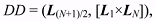(1)

where Lj is the unit vector (cosαj cosδj, sinαj cosδj, sinδj).

If DD is equal to 10-6 ÷ 10-5 , then the accuracy loss will be close to 5-6 digits; especially, in the following orbital elements: the semi-major axis (a), the eccentricity (e), and the argument of the perigee (ω). So the value of DD can be used as an indicator of the resulting reliability.

However, the preliminary values of topocentric distances can be calculated by using both the orbital inclination (i) and the longitude of the ascending node (Ω) of the circular orbit, and the conditions :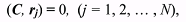(2)

where C = (sini sinΩ, –sini cosΩ, cosi) is the unit vector perpendicular to the orbital plane, and rj, the vector of the geocentric distance of the object for the time-moment tj of observation. This is expressed as follows: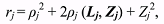(3)

or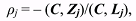(4)

where Zj is the geocentric vector of an observatory with coordinates (Xj, Yj, Zj), calculated for corresponding time-moments tj. Then the equation for ρj can be obtained by multiplying equation (3) by the vector С and taking into account (2):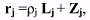(5)

By use of formulae (3) (5) the geocentric rectangular coordinates xj, yj, zj of GO for each time-moment of observations, the distances rj and the arguments of latitude uj = νj + ω (where νj is the true anomaly) can be computed by use of the formulae of the two-body- problem. The obtained rj and uj can be represented by Taylor series as follows: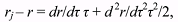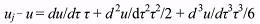(6)

where r and u denote the values of rj and uj for the initial moment of time t0, and τ = GM (t–t0) (GM = 107.08828 in terms of the constants adopted for the GO).

The analytical representations of the derivatives in terms of the orbital elements are given as follows: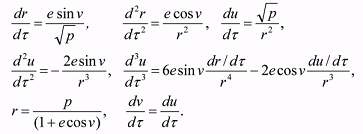(7)

It should be noticed that for circular orbits the first derivatives dr/dτ = 0 and (du/dτ) 3r = 1, but the second derivatives are close to their exact values. Taking into account the analytical expressions (7) of the derivatives, the following expression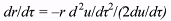(8)

can be used in the second approximation instead of dr/dτ.

The equation (8) allows organizing the iteration process, which is shown in Figs. 1 and 2.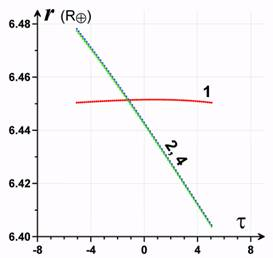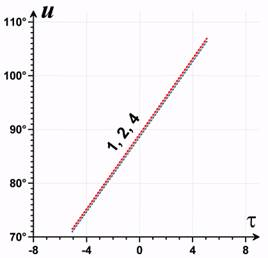Fig. 1. The representation of several approximations for rj Fig. 2. The representation of several approximations for uj.

After calculating more precise rj and uj in the second approximation all orbital elements are determined. The main idea of the method is to achieve an agreement between the elements, which are calculated from the geometry (by use of rj), and those from the dynamics (by use of uj).
##### 2. THE CHECK CALCULATIONS

To check the method the model observations have been generated using the GO 68081E fragment data obtained by V. Biryukov and V. Rumyantsev at the Crimean observatory. From the beginning its orbit was improved, all perturbations being taken into account, by use of the observations for October 18, 2004, distributed over the 160 minute time interval. After that the ephemerides were calculated for 69 points with the step of 2 minutes, taking into account the secular perturbations only.

In Table 1 in the first line the improved values of the orbit elements are given. In the second line the elements of the preliminary orbit are shown, calculated by the new method, by use of all 69 model observations. The determinant of the system DD = 0.003571, and the obtained orbit slightly differs from the initial one, representing the model observations to the precision of 0”.06.

Table 1. The elements of preliminary orbits, calculated by use of the model observations.

T0(MJD) e i Ω ω M n λ dλ/dt σ
53296.90625 0.01970 11°.448 338°.433 195°.682 237°302 1.05045 57°.452 17.2104°/day 0".6
53296.95347 0.01961 11.469 338.544 195.507 255.221 1.05054 58.261 17.2430°/day 0".06

The representation errors for the model observations on short time-intervals are smaller than 0”.1. The errors of representation by means of circular orbits are 1-3”.

In Table 2 eight orbits are shown as calculated on the short time-interval by use of the same observations.

Table 2. Preliminary orbits calculated by use of model observations over the short time intervals (?t = 16 minutes) and with the determinants DD, which are 0.579015x10-5 through 0.609425x10-5.

T0(MJD) e i Ω ω M n λ σ
53296.91181 0.01994 11.°470 338.°555 194.°895 240.°109 1.05050 57.°589 0".06
53296.92431 0.01930 11.468 338.542 194.470 245.218 1.04962 57.746 0".02
53296.93681 0.01974 11.469 338.546 195.850 248.582 1.05087 57.983 0".13
53296.94931 0.01963 11.469 338.547 194.847 254.314 1.05010 58.200 0".10
53296.96181 0.01936 11.469 338.536 196.171 257.683 1.05090 58.371 0".07
53296.97431 0.01928 11.470 338.534 196.713 261.860 1.05131 58.575 0".07
53296.98681 0.01992 11.468 338.553 194.795 268.569 1.05004 58.873 0".11
53296.99653 0.01980 11.469 338.550 195.518 271.511 1.05053 59.024 0".05

The errors of representation of the model observations on these short time-intervals are smaller than 0”.2. The errors of representation by means of circular orbits are 1-3”.

In Table 3 the preliminary orbits of GO 90003 are shown as having been calculated by use of the real observations for different time-intervals, their different distributions being given. The letter “с” means a circular orbit and “е” means an elliptic one.

In the first case the determinant DD = 0.17063x10-5 and the precision of the observations is 10 times worse than in the case of the model observations. Therefore, the values of elements e, ω and n (the mean motion of the object) differ from the check orbit, and it is necessary to extend the time-interval in order to increase the precision of these elements.

Table 3. Preliminary orbits calculated by use of the real observations of GO 90003.

 DD = 0.17063x10-5 0 Date 2004/10/18.9378238 N=9 Δt = 11min N T0 α δ (O-C)c (O-C)e 1 22h24m27s.970 4h08m29s.960 5°41'18".660 -".12 ".26 ".04 1".07 2 22 25 37.970 4 09 44.210 5 41 52.630 1.47 1.25 -.57 -.05 3 22 26 47.970 4 10 58.550 5 42 26.660 1.78 .89 -.10 .18 4 22 27 57.970 4 12 12.880 5 42 59.230 2.29 .69 -.05 .27 5 22 30 27.980 4 14 52.220 5 44 04.570 2.82 .38 -.17 .41 6 22 31 37.970 4 16 06.660 5 44 33.270 1.71 -.02 .71 .75 7 22 32 47.980 4 17 20.970 5 44 59.840 2.89 .43 -1.01 .27 8 22 33 58.110 4 18 35.610 5 45 25.320 1.10 .72 -.07 -.03 9 22 35 09.130 4 19 51.160 5 45 50.540 -.12 .26 -.07 .43 n e i Ω ω λ σ c 1.03582 .00000 11.425 337.931 .000 55.896 1" 4 Check .01970 11.448 338.433 195.682 e 53296.93782 .03486 11.489 338.463 228.350 58.191 0".681 n = 1.09495 DD = 0.674864x10-4 0 Date 2004/10/18.9621425 N=9 Δt = 47.5min N T0 α δ (O-C)c (O-C)e 1 22h59m39s.000 4h45m58s.870 5°49'19".540 -".13 ".27 -".63 1".77 2 23 03 09.000 4 49 43.500 5 49 00.740 14.14 1.21 .36 .38 3 23 04 19.000 4 50 58.350 5 48 51.960 19.24 1.38 .21 .07 4 23 05 29.110 4 52 13.360 5 48 43.100 23.68 .27 .39 1.01 interval 37min 5 23 42 05.500 5 31 30.580 5 32 19.820 20.25 -1.51 .21 1.16 6 23 42 56.170 5 32 25.080 5 31 41.220 17.27 -.88 .10 .67 7 23 43 47.130 5 33 19.790 5 31 02.370 15.73 -.90 -1.59 .86 8 23 45 27.110 5 35 07.420 5 29 43.810 8.14 -.59 -.73 .91 9 23 47 10.500 5 36 58.730 5 28 19.260 -.13 .27 .05 .49 n e i Ω ω λ σ c 1.0457138 .00000 11.460 337.877 .000 56.201 11".3 Check .01970 11.448 338.433 195.682 e 53296.96214 .02161 11.454 338.468 203.634 58.522 1".1 n = 1.05728 DD = 0.797428x10-4 0 Date 2004/10/18.9198029 N=36 Δt = 48min N T0 α δ (O-C)c (O-C)e 1 21h45m02s.980 3h26m52s.660 5°09'17".750 -".11 ".26 ".84 ".31 ............................................................ 36 22 23 17.970 4 07 15.650 5 40 41.670 -.12 .25 .07 -.5 n e i Ω ω λ σ c 1.03159 .00000 11.410 337.966 .000 55.709 16". 3 Check .01970 11.448 338.433 195.682 e 53296.91980 .02069 11.453 338.417 201.434 57.654 0".77 n = 1.05523
##### 3. CONCLUSIONS

In conclusion it should be noted that the suggested method of orbit determination for the geostationary objects permits achieving, as compared with classical methods, a better precision on the short time-interval.

Acknowledgements. This research is supported by the Grant of INTAS-01-0669.
##### REFERENCES

1. Schildknecht T., R. Musci, W. Flury, J. Kuusela, J. de Leon, L. de Fatima Dominguez Palmero, Optical observations of space debris in high-altitude orbits, 4th European Conference on Space Debris, April 18-20, 2005, ESOC, Darmstadt, Germany (in press).
2. Субботин М. Ф., Введение в теоретическую астрономию, М., 1968, 800 с.

Размещен 6 декабря 2006.